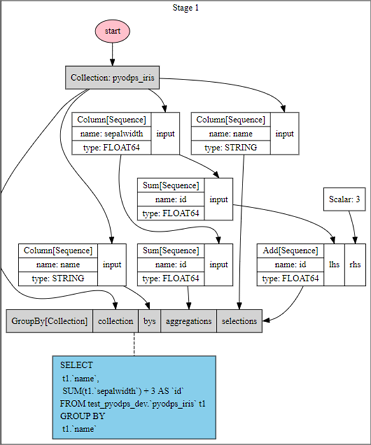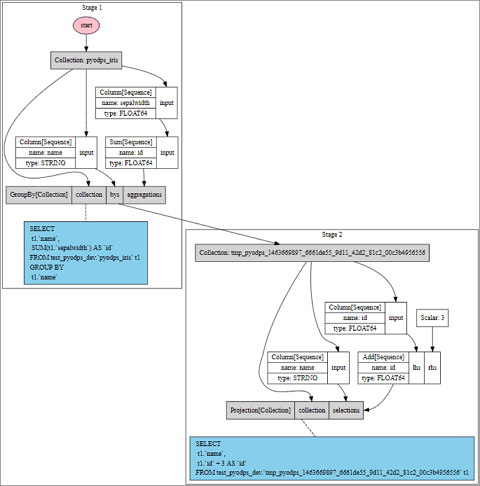## 可视化DataFrame

``````>>> df = iris.groupby('name').agg(id=iris.sepalwidth.sum())
>>> df = df[df.name, df.id + 3]
>>> df.visualize()````````````>>> df = iris.groupby('name').agg(id=iris.sepalwidth.sum()).cache()
>>> df2 = df[df.name, df.id + 3]
>>> df2.visualize()``````## ODPS SQL后端查看编译结果

``````>>> df = iris.groupby('name').agg(sepalwidth=iris.sepalwidth.max())
>>> df.compile()
Stage 1:

SQL compiled:

SELECT
t1.`name`,
MAX(t1.`sepalwidth`) AS `sepalwidth`
FROM test_pyodps_dev.`pyodps_iris` t1
GROUP BY
t1.`name```````

## 使用Pandas计算后端执行本地调试

• 非分区表：对其进行选取全部或有限条数据、列筛选的操作（不包括列的各种计算），以及计算其数量。
• 不选取分区或筛选前几个分区字段的分区表：对其选取全部或有限条数据、列筛选的操作，以及计算其数量。

``````>>> iris.count()
>>> iris['name', 'sepalwidth'][:10]``````

``````>>> df[:10]
>>> df[df.ds == '20160808']['f0', 'f1']
>>> df[(df.ds == '20160808') & (df.hh == 3)][:10]
>>> df[(df.ds == '20160808') & (df.hh == 3) & (df.mm == 15)]``````

``````>>> DEBUG = True
>>> if DEBUG:
>>> df = iris[:100].to_pandas(wrap=True)
>>> else:
>>> df = iris``````